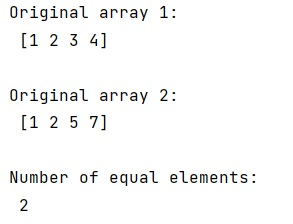# Check how many elements are equal in two numpy arrays?

Learn, how to check how many elements are equal in two numpy arrays in Python?
Submitted by Pranit Sharma, on January 23, 2023

NumPy is an abbreviated form of Numerical Python. It is used for different types of scientific operations in python. Numpy is a vast library in python which is used for almost every kind of scientific or mathematical operation. It is itself an array which is a collection of various methods and functions for processing the arrays.

## NumPy - Checking how many elements are equal in two arrays

Suppose that we are given two NumPy arrays and we need to check how close these two NumPy arrays are that means how many values are equal in these two NumPy arrays.

There is an easy approach to this problem. If two arrays have an equal number of elements and if all the elements of both arrays are equal, then the sum of one array must be equal to the sum of another array.

But for this purpose, we will apply a condition that if an element of both arrays is equal, it is added to the total sum of the equal elements.

Let us understand with the help of an example,

## Python code to check how many elements are equal in two numpy arrays

```# Import numpy
import numpy as np

# Creating two numpy arrays
arr1 = np.array([1, 2, 3, 4])
arr2 = np.array([1, 2, 5, 7])

# Display original arrays
print("Original array 1:\n",arr1,"\n")
print("Original array 2:\n",arr2,"\n")

# Finding total number of equal elements
res = np.sum(arr1==arr2)

# Display the result
print("Number of equal elements:\n",res)
```

Output: